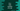# C++ program to find and print all subsets of a set## C++ program to print all subsets of a set:

In this C++ program, we will learn how to print all subsets of a set. The program will take the set elements as inputs from the user and it will print all subsets of that set.

We will use an integer array in the example but you can also modify it to use any other type of array. The program can handle variable array sizes.

### Algorithm:

Before moving to the algorithm, let’s say we want to print the subsets for the set {1, 2, 3}.

Following are all possible subsets of this set:

``````{}
{1}
{2}
{1, 2}
{3}
{1, 3}
{2, 3}
{1, 2, 3}``````

For a set of size n, we can generate 2^n number of subsets, where n is the size of the set.

We will use a process using bit-masking to print all the possible subsets. Below is the complete algorithm:

• Get the value of n as an input from the user.
• Take all n values of the set and insert the values to an array.
• We can generate 2^n number of binary numbers of size n.
• For each binary number, we need to check which bit is set. For the set bit position, include the element from the array of that position in the subset.

#### Examples:

Let’s take an example for set {1, 2}.

• It has 2 elements. So, we can create 2^2 = 4 binary numbers of size 2.
``````00
01
10
11``````

For each binary number, we will check the set bits, and we will pick the element from the set for a set bit position.

• 00 => {} (no bit is set)
• 01 => {2} (only the bit at second position is set, so include only the second element)
• 10 => {1} (only the first bit is set, so include only the first element)
• 11 => {1, 2} (both bits are set, include both elements)

Let’s take another example for set {2, 3, 4}.

• It has 3 elements. So, we can create 2^3 = 8 binary numbers of size 3.
``````000
001
010
011
100
101
110
111``````

So, let’s create the subsets:

• 000 => {}
• 001 => {4}
• 010 => {3}
• 011 => {3, 4}
• 100 => {2}
• 101 => {2, 4}
• 110 => {2, 3}
• 111 => {2, 3, 4}

### C++ program:

Let’s write down the program in C++:

``````#include <iostream>
#include <string>
#include <math.h>

using namespace std;

void printSubSet(int s[], int size)
{
string subSet;

for (int i = 0; i < pow(2, size); i++)
{
subSet = "{";
for (int j = 0; j < size; j++)
{
if ((i & (1 << j)) != 0)
subSet += to_string(s[j]) + ",";
}

if (subSet.length() > 2)
{
subSet = subSet.substr(0, subSet.size() - 1);
}

subSet += "}";

cout << subSet << endl;
cout << "\n";
}
}

int main()
{
int size;

cout << "Enter the size:" << endl;
cin >> size;

int s[size];
cout << "Enter the numbers of the set one by one:" << endl;

for (int i = 0; i < size; i++)
cin >> s[i];

cout << "\nSubsets of this set are: " << endl;
printSubSet(s, size);

return 0;
}``````

Here,

• It asks the user to enter the size of the set and stores that value in the size variable.
• It creates an array s to hold the set elements. By using a for loop, it takes the set elements from the user and inserts these to the array s.
• The printSubSet method is used to print all the subsets.
• It uses two for loops. The outer loop runs for 2^size number of times.
• The inner loop finds the set bits and if any bit is set, it adds that to subSet.
• subSet is a string variable to create the subset. It adds all numbers separated by ,.
• Once the inner for loop is done, we are removing the last extra comma from subSet if there is any.
• Finally, the value of subSet is printed, which is the subset for the current iteration.

It will give output as like below:

``````Enter the size:
3
Enter the numbers of the set one by one:
2
3
4

Subsets of this set are:
{}

{2}

{3}

{2,3}

{4}

{2,4}

{3,4}

{2,3,4}``````